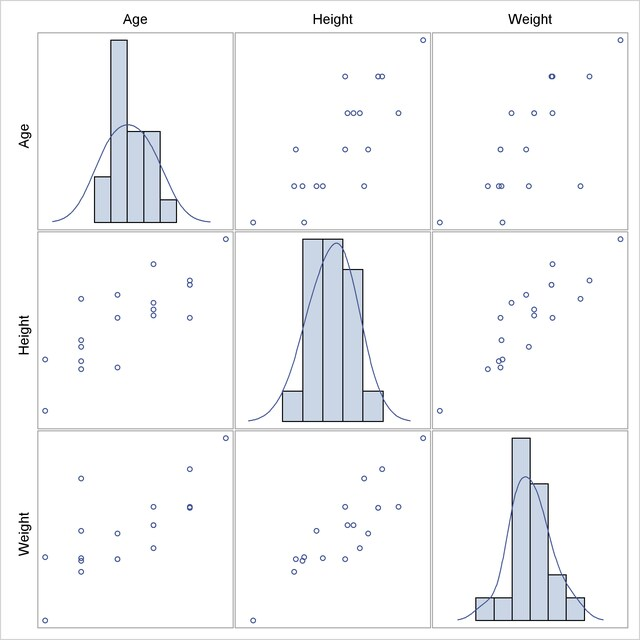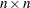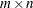Statistical Graphics Using ODS
 The SGSCATTER Procedure

You can use the SGSCATTER procedure to produce scatter plot matrices. The following step creates a scatter plot matrix from all of the numeric variables in the Class data set available in the SASHELP library and produces Figure 21.37:

```proc sgscatter data=sashelp.class;
matrix _numeric_ / diagonal=(kernel histogram);
run;
```

The diagonal cells of Figure 21.37 contain a histogram and a kernel density fit. The off-diagonal cells contain all pairs of scatter plots.

Figure 21.37 Scatter Plot Matrix with PROC SGSCATTERThe MATRIX statement creates a symmetricscatter plot matrix. Other statements are available as well. The PLOT statement creates a panel containing one or more individual scatter plots. The COMPARE statement creates a rectangularscatter plot matrix. Linear and nonlinear fits can be added, and many graphical features can be requested with options.Previous Page | Next Page | Top of Page